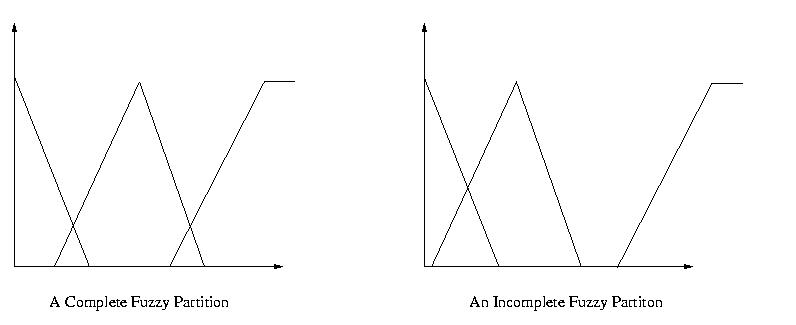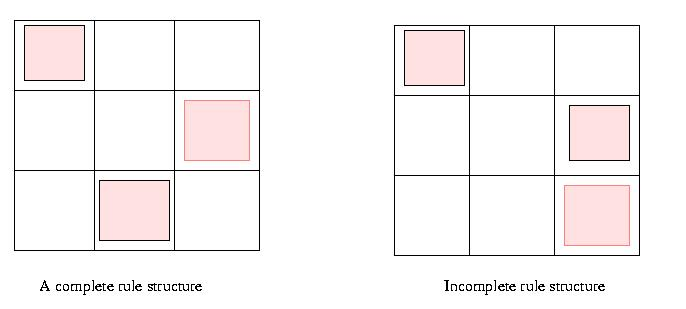### Generating Complete, Distinguishable, Consistent and Compact Fuzzy Systems Using Evolutionary Algorithms

#### Completeness

Completeness of fuzzy systems consists of two main factors: completeness of fuzzy partitions and completeness of fuzzy rule structure. Example of complete/incomplete fuzzy partion, as well as complete/incomplete fuzzy rule structure are shown in Fig.1 and Fig. 2.Fig. 1

#### Distinguishability

Distinguishability is one of the most important aspect for interpretability of fuzzy systems. It is possible to assign a linguistic term to a fuzzy subset only of this condition is satisfied. Fig. 2 shows a distinguishable and an indistinguishable fuzzy partiton.Fig. 2

The completeness and distinguishability of a fuzzy partition can be guaranteed by imposing a constraint on the similarity between two neighbouring fuzzy subsets.

s1 < S(A,B) < s2,

where 0 < s1 < s2 < 1, S(A,B) is a fuzzy similarity measure. Notice that S(A,B) =0 means that the two fuzzy subsets do not overlap and S(A,B) = 1 that the two fuzzy subsets are the same. Both situation should be avoided. In fact, S(A,B) should be siginificantly less than 1 so that A is distinguishable.

#### Consistency of Fuzzy Rules

Fuzzy rules should be consisteny with each other and consistent with human heuristics (a priori knowledge). Intuitively, if the condition part of two fuzzy rules are similar, then the consequent part of the two rules should also be similar. A consistency definition based on this heuristics has been suggested in .

#### Compactness

Compactness of fuzzy systems includes two aspects: a small number of conditions in the rule premise and a small number of fuzzy rules in the rule base.

A method considering these interpretability conditions in addition to approximation performance has been proposed in  using evolution strategies to generate interpretable fuzzy rules from data.

#### References

 Y. Jin, W. von Seelen and B. Sendhoff. On generating flexible, complete, consistens and compact (FC3) fuzzy rules from data using evolution strategies. IEEE Transactions on Systems, Man, and Cybernetics, 29(4):829-845, 1999